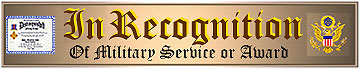Operation Tidal Wave
Display Recognition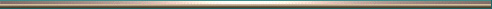Site Index

• Example Display Recognition
• Applicable Military Branches
• Display Types
• Issue Requirements
• Issue Regulations
• Who May Apply
• Administration Processing and Delivery
• How To Apply For This Display Recognition and/or Medal
• Mailing Address
• Questions?
• Other Display Recognitions
• Air Service Websites
•Example Display Recognition:
Actual Size: 8 1/2 x 11Military Branches

All Military Service branches are applicable:
Air Force, Army, Coast Guard, Marine Corps, NavyWho May Apply

• Veteran recipients
• Active Duty recipients
• Family Members of recipients
• Estate InheritorsDisplay Types

You have four display types to choose from:
Black & White (No Color)
Heavy Bond Enclosure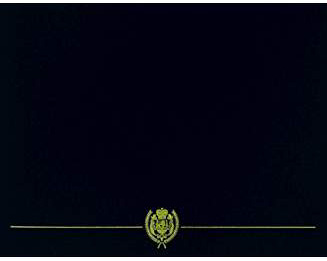Heavy Bond Enclosure
Colors: Regal, Black, Navy
Trimmed in Appearance Gold

Ready for Mantle or Shelf DisplayIssue Requirements
You must submit the following:

• An unaltered photocopy of your Report of Separation clearly displaying your Army Air Force service in the EAME Theater during the Mission Period dates.
•  NOTICEThis Display Recognition is available ONLY to authorized recipients who possess orders, or authorization form, or release documentation that confirms award eligibility. (See "Issue Requirements"). To obtain either a Display Medal or a Display Recognition for your authorized award you will be required to provide military-issued documentation authorizing your award. There are no exceptions. WARNING!You must NOT submit a military issued document or photocopy that:has been altered in any way by you afterthe original's official issuecontains information or corrections oradditions that you enteredlists awards or training you knowingly did not receivecontains highlighting, colorizing or other markings you entered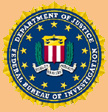Be advised that on request any knowingly fraudulent document sent by you will be released to the Federal Bureau of Investigation (FBI) that may result in prosecution and/or fine/imprisonment. Email Questions, or Phone: 1-562-422-4100 (Pacific Time Zone)Personnel Branch
Processing and Delivery

Style Admin Shipping Total
Full Color .PDF (Printable) \$7.50 Emailed \$7.50
Published Document only (Full Color) \$14.00 \$9.90 \$23.90
Published Document in Heavy Bond Enclosure (Full Color) \$19.00 \$9.90 \$28.90
Full Color Printable Certificates
on CD-Rom (minimum 20 Certificates)
\$7.50 \$7.50 By Count

• *** USPS USPS Shipping fee based on 1 Certificate shipped to any U.S. state, FPO or APO
• ** USPS USPS Shipping fee based on 1 Certificate shipped to any U.S. state, FPO or APO
• Contact The War Library for international shipping cost
•How to Apply

You may apply for your Display Recognition using a...

• Check Debit card (* Shipped within 1 week)
• Charge card (* Shipped within 1 week)
• Personal, Business, Bank or Cashiers Check ( Shipped in 6 weeks)
• Money Order (* Shipped in 6 weeks)
• (* Upon reception of required documentation)

Complete and MAIL this APPLICATION FORM.
Don't forget to include an unaltered COPY of your DD-214, WD AGO 53-55 or other pre-arranged document(s).

DISPLAY RECOGNITION and/or MEDAL APPLICATION FORMMailing Address

Records Chief (REC-AMW)
The American War Library
817 East Via Carmelitos Ste 319
Long Beach CA 90805-7549

Click here for answers to common questions

Email: Operation Tidal Wave Display Recognition Inquiry

Telephone: 1-562-422-4100 (Pacific Time Zone)Copyright ©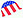The American War Library
Military and Veteran Websites
The G.I. Photograph Museum of Honor
Locator Registry Applications
Accessing The Worldwide Military Personnel DatabaseIssue Regulations
Operation Tidal WaveClick for larger image

Authorized For Army Air Force Service in the European, African, Middle-Eastern Theater During the Mission Period: 1 August 1943 To 3 August 1943

Operation Tidal Wave involved a commitment of one hundred seventy eight B-24 Liberator heavy bombers on a twenty-four hundred mile round trip flight to conduct low-level attack on the Ploesti Oil fields in Romania operated by Germany to fuel its continental war machine. Although this essential operation was one of the most costly in terms of aircraft and crew members lost by the United States Army Air Forces during the strategic bombing campaign over Europe its necessity was most urgent. Among the heroism displayed the Mission resulted in the award of five Medals of Honor. Service During this Missions Period was instrumental in the Overall Success of our Victory over Axis Forces.Plane Number/Name/Pilot/Unit/Status in mission

• 41-24192=DOGPATCH RAIDER=Avandano Joseph=328thBS=Diverted to Sicily=
• 41-11776=JERSEY JACKASS/BASHFUL=McGraw John J=389thBG=Lost near the target Ploesti=
• 41-11620=EDNA ELIZABETH=Haverty James C=93rdBG=Aborted=
• 41-11761=THE SQUAW/SLEEPY=LeBrecht Royden L=343thBS=Diverted to Cyprus=
• 41-11766=CHUG-A-LUG=Morgan LeRoy B=345thBS=Returned to Libya=
• 41-11767=SHANGHI LIL=Looker Carl S=343thBS=Aborted=
• 41-11768=KICKAPOO=Nespor Robert J=344thBS=Aborted=
• 41-11774=CHIEF=Fravega Thomas P=389thBG=Diverted to Sicily=
• 41-11803=ROSIE WRECK'EM=Arens Herbert W=344thBS=Aborted=
• 41-11815=DAISY MAE=Ellis Lewis N=389thBG=Returned to Libya=
• 41-11817=STINGER=Opsata Andrew W=389thBG=Returned to Libya=
• 41-11819=RAUNCHY=Neeley Samuel R=344thBS=Lost near the target Ploesti=
• 41-11825=Hail Columbia/LITTLE-CHIEF BIG-DOG=Kane John Riley=98HQ=Lost -- Crash landed on Cyprus=
• 41-11836=LI'L DE-ICER=Merrick James L=415thBS=Returned to Libya=
• 41-11840=THE WITCH=Darlington Julian T=389thBG=Lost -- crash land at Bulgaria-Yugoslavia border=
• 41-11886=LIL JOE=Hussey Lindley P=345thBS=Lost near the target Ploesti=
• 41-11896=U-=NIGHTMARE=Bleyer Julian N=98HQ=Returned to Libya=
• 41-11921=NORTHERN STAR/DOC=Underwood Glen W=343thBS=Returned to Libya=
• 41-23656=ROWDY II=Gaston Allen B=344thBS=Aborted=
• 41-23661=BLACK JACK=Hahn Delbert H=345thBS=Diverted to Sicily=
• 41-23682=THE BLASTED EVENT=Brown George S=93HQ=Returned to Libya=
• 41-23699=LEMON DROP=Phillips Reginald H=68thBS=Returned to Libya=
• 41-23711=JERKS NATURAL=Hickman Cleveland D=328thBS=Diverted to Sicily=
• 41-23717=EXTERMINATOR=Roper Hugh R=330thBS=Lost -- Mid-air collision with Olliffe during withdrawl=
• 41-23722=BOMERANG=Martin Roy G=328thBS=Returned to Libya=
• 41-23729=SHOOT LUKE=Black George C=328thBS=Aborted=
• 41-23733=SKIPPER=Colchagoff George D=344thBS=Aborted=
• 41-23742=LIBERTY LAD=McFarland Kenton D=409thBS=Returned to Libya=
• 41-23754=LITTLE LADY=Wilkinson Richard L=409thBS=12=12=Lost -- Landed at Chorlu Turkey; Interned=
• 41-23778=LADY LUCK=Felber Robert E=66thBS=Returned to Libya=
• 41-23779=4Q2=Henderson Charlie P=67thBS=Diverted to Malta=
• 41-23782=BOILER MAKER II=Helin Theodore E=415thBS=10=Lost near the target Ploesti=
• 41-23788=AVENGER=Hughes William D=68thBS=Returned to Libya=
• 41-23795=SNEEZY=Johnson Donald G=343thBS=Returned to Libya=
• 41-23809=HELLSADROPPIN II=Harvey Julian A=329thBS=Aborted=
• 41-23810=TARFU=Hurd Earl C=329thBS=Returned to Libya=
• 41-23811=FASCINATIN' WITCH=Miller Robert E=66thBS=Diverted to Malta=
• 41-23813=V=VICTORY SHIP=Diehl John H=68thBS=Returned to Libya=
• 41-23817=SUZY~Q=Brandon William H=44HQ=Returned to Libya=
• 41-23936=MARGURITE/QUEEN ANNE=Flaherty Joseph E=66thBS=Returned to Libya=
• 41-24015=WING DINGER=Winger George W=66thBS=Lost near the target Ploesti=
• 41-24023=FERTILE MYRTLE=Shingler Herbert I=415thBS=Returned to Libya=
• 41-24024=34=BEWITCHING WITCH=Carpenter Reginald L=67thBS=Lost -- Ditched in Med. On return flight=
• 41-24026=BABY=Weisler Francis E=344thBS=Aborted=
• 41-24040=BIG OPERATOR=Edwards Hoover=344thBS=Aborted=
• 41-24105=TUPELO LASS=Dessert Kenneth O=409thBS=Returned to Libya=
• 41-24109=READY & WILLING=Roche John R=330thBS=Returned to Libya=
• 41-24111=PICCADILLY FILLEY=Collins Theron U=329thBS=Aborted=
• 41-24147=THE DUCHESS/EVELYN=Potts Ramsay D=330thBS=Returned to Libya=
• 41-24153=SAD SACK II=Lasco Henry A=66thBS=Lost near the target Ploesti=
• 41-24195=LITTLE JOE=Pettigrew Wesley N=415thBS=Returned to Libya=
• 41-24197=TAGALONG=Hinch Ralph V=344thBS=Lost near the target Ploesti=
• 41-24198=THE VULGAR VIRGIN=Taylor Wallace C=344thBS=Lost near the target Ploesti=
• 41-24201=O=BALDY AND HIS BROOD=Strong William H=506thBS=Returned to Libya=
• 41-24211=WING AND A PRAYER=Holmes Walter T=68thBS=Returned to Libya=
• 41-24215=LUCKY=Kendall Harold K=409thBS=Diverted to Sicily=
• 41-24225=FLAK ALLEY=Alexander David W=68thBS=Returned to Libya=
• 41-24226=UTAH MAN=Stewart Walter T=330thBS=Returned to Libya=
• 41-24229=BUZZIN' BEAR=Cameron William R=67thBS=Returned to Libya=
• 41-24230=Jo-Jo'S Special Delivery/Hare's to Ya=McBride Ralph J=329thBS=11=Lost -- Shot down over Ionian Sea on return flight=
• 41-24232=CALABAN=Hill James E=67thBS=Diverted to Malta=
• 41-24234=MR. 5 X 5=Beam James C=506thBS=Returned to Libya=
• 41-24235=EARTHQUAKE (MCGOON)=Bunker Walter I=506thBS=Returned to Libya=
• 41-24246=SEMPER FELIX=Sulflow August W=345thBS=Lost near the target Ploesti=
• 41-24259=30=unknown if any=Freese Howard W=329thBS=Aborted=
• 41-24282=RUTH-LESS=Slough Frank D=506thBS=Returned to Libya=
• 41-24283=OLD CROW=McAtee James C=506thBS=Returned to Libya=
• 41-24297=20-E=CHIEF WAHOO=Hines Wilford E=512thBS=10=Lost -- Landed Kardak LG Turkey; Interned=
• 41-24298=QUEENIE=Brown Llewellyn L=409thBS=Diverted to Sicily=
• 41-24311=HADLEY'S HAREM=Hadley Gilbert B=344thBS=Lost -- ditched off south coast of Turkey=
• 42-24121=LET'ER RIP=Olliffe Victor E=330thBS=Lost -- Mid-air collision with Roper during withdrawl=
• 42-40071=MARGARET ANN II=Jansen George R=68thBS=Returned to Libya=
• 42-40082=PRINCE CHARMING=Bennett Thomas W=343thBS=Aborted=
• 42-40102=OLD BALDY=Dore John J=345thBS=10=Lost near the target Ploesti=
• 42-40127=THAR SHE BLOWS AGAIN=Merrill Charles T=329thBS=Returned to Libya=
• 42-40128=BALL OF FIRE III/WAR BABY=Tate Joseph S=328thBS=Returned to Libya=
• 42-40172=LYNN BARI II=Anderson William N=506thBS=Returned to Libya=
• 42-40182=FORKY II=Gentry Rowland M=66thBS=Lost near the target Ploesti=
• 42-40195=PENELOPE=Speiser Martin A=415thBS=Returned to Libya=
• 42-40208=SAD SACK=Banks William D=344thBS=Diverted to Cyprus=
• 42-40246=THUNDER MUG=Longnecker Russell D=328thBS=Returned to Libya=
• 42-40265=HONKEY TONK GAL=Womble Hurbert H=409thBS=Lost near the target Ploesti=
• 42-40267=HORSE FLY=Mitchell Edward R=67thBS=Lost -- Landed at Chorlu Turkey; Interned=
• 42-40312=AIRE LOBO=Thomas John B=345thBS=Lost near the target Ploesti=
• 42-40313=BOOTS=Murphy Lawrence E=343thBS=Lost near the target Ploesti=
• 42-40316=SNAKE EYES=Blevins Hillary M=345thBS=Lost -- Crash landed on Sicily=
• 42-40322=CORNHUSKER=McCarty Ned=415thBS=10=Lost -- Shot down over Ionian Sea on return flight=
• 42-40364=SNOW WHITE and the Seven Dwarves=Gunn James A. III=343thBS=Lost over Bulgaria on return flight=
• 42-40370=HEAVEN CAN WAIT=Whitlock Charles A=506thBS=Aborted=
• 42-40371=G.I. GAL=Reinhart Elmer H=67thBS=Lost over Romania on return flight=
• 42-40373=Z=NATCHEZ BELLE=Shannon Eunice M=68thBS=Returned to Libya=
• 42-40375=SCRAPPY II=Scrivner Thomas E=66thBS=Lost near the target Ploesti=
• 42-40402=THE SANDMAN=Sternfels Robert W=345thBS=Diverted to Cyprus=
• 42-40520=YEN TU=McGuire Edward T=415thBS=Lost near the target Ploesti=
• 42-40604=SATAN'S ANGELS=Ford Herrel E=328thBS=Returned to Libya=
• 42-40606=TIMBA-A-H!=Larson Richard A=506thBS=Returned to Libya=
• 42-40608=VULGAR VIRGIN=Turner Claude A. >Turner Claude A=328thBS=10=Lost -- Landed at Chorlu Turkey; Interned=
• 42-40609=JERSEY BOUNCE=Long Worthy A=330thBS=Lost near the target Ploesti=
• 42-40610=unknown if any=League Miles R=409thBS=Returned to Libya=
• 42-40611=DEATH DEALER=Geron Alva J=409thBS=Returned to Libya=
• 42-40612=EUROCLYDON THE STORM=Porter Enoch M=328thBS=Lost near the target Ploesti=
• 42-40613=PUDGY=Teltser Milton=330thBS=Lost over Romania on return flight=
• 42-40617=JOSE CARIOCA=Stampolis Nicholas=409thBS=10=Lost near the target Ploesti=
• 42-40655=DAMIFINO or FOUR EYES=Hadcock Lawrence=343thBS=Lost near the target Ploesti=
• 42-40662=BLACK MAGIC=Patch Dwight D=415thBS=Returned to Libya=
• 42-40663=MATERNITY WARD=Ward John V=345thBS=Lost -- Shot down over Ionian Sea on return flight=
• 42-40731=HAG MAG the Mothball Queen=Martin George P=68thBS=Returned to Libya=
• 42-40746=OLE IRISH=McLaughlin Frank B=564thBS=Returned to Libya=
• 42-40764=HELEN B. HAPPY=Irby Shelby L=66thBS=Returned to Libya=
• 42-40765=VALIENT VIRGIN=DeMont Russell D=329thBS=Returned to Libya=
• 42-40777=FLOSSIE FLIRT=Hughes Charles E=66thBS=Lost -- Landed at Chorlu Turkey; Interned=
• 42-40778=T=SOUTHERN COMFORT=Austin Horace W=506thBS=Returned to Libya=
• 42-40780=AVAILABLE JONES=Jones Fred E=67thBS=Lost -- Shot down over Ionian Sea on return flight=
• 42-40781=HELLS ANGELS=Harms Roy C=329thBS=Lost near the target Ploesti=
• 42-40804=THE LADY JANE/BERTHA=Meehan William E=329thBS=Lost near the target Ploesti=
• 42-40969=BIG NOISE=Emmons John W=330thBS=Aborted=
• 42-40973=BATTLEAXE=Salyer Charles A=389thBG=Returned to Libya=
• 42-40991=KATE SMITH=Deeds James A=345thBS=Lost near the target Ploesti=
• 42-40994=HELL'S WENCH=Baker Addison E. >Baker Addison E=93HQ=10=Lost near the target Ploesti=
• 42-40995=SATAN'S HELL CATS=Houston Rowland B=67thBS=Lost over Romania on return flight=
• 42-41007=MARGIE=Gooden Clarence W=343thBS=Lost near the target Ploesti=
• 42-41013=TROUBLE=Stevens Gordon S=506thBS=Returned to Libya=
• 42-63758=LI'L JUGHAID=Nicholson Robert G=415thBS=Returned to Libya=
• 42-63761=LIL' ABNER=Weaver Worden L=67thBS=Lost over Romania on return flight=
• 512=41-23724=22-Z=DOODLEBUG=McAtee John E=512thBS=Returned to Libya=
• 512=41-24258=23-A=DESERT LILLY=Iovine Guy T=512thBS=Aborted=
• 512=41-24294=36-V=BREWERY WAGON=Palm John D=512thBS=Lost near the target Ploesti=
• 512=42-40229=31-B=BOMB BOOGIE=Miller Richard T=512thBS=Returned to Libya=
• 512=42-40563=28-D=WONGO WONGO!=Flavelle Brian W=512thBS=10=Lost -- Crashed into the Med at 1130 south of Corfu=
• 512=42-40658=38-F=WARMAID=Miller James I=512thBS=Diverted to Cyprus=
• 513=41-11591=45-G=QUEEN BEE/LORRAINE=Zimmerman William R=513thBS=Returned to Libya=
• 513=41-11779=41-N=LI'L ABNER=Whitlock Hubert H=513thBS=Returned to Libya=
• 513=41-11916=42-F=TANGERINE=Hobbs Charles L=513thBS=Returned to Libya=
• 513=41-24032=51-K=LET'S GO!=Fogel Ernest W=513thBS=Returned to Libya=
• 513=42-40315=40-P=WOLF PACK=DeVeuve James P=513thBS=Returned to Libya=
• 513=42-40317=54-L=BARREL HOUSE BESSIE=Friberg Wayne V=513thBS=Returned to Libya=
• 513=42-40660=56-A=LITTLE RICHARD=Lear Dean E=513thBS=Returned to Libya=
• 514=41-11636=63-P=WASH'S TUB=Bock James=514thBS=Returned to Libya=
• 514=41-11935=60-E=PER DIEM THE SECOND=Storz Robert H=514thBS=Returned to Libya=
• 514=41-23410=72-J=unknown if any=Whipple Harlan D=514thBS=Returned to Libya=
• 514=41-24252=70-W=Ryan Lyle T=514thBS=Returned to Libya=
• 514=41-24310=62-D=unknown if any=O'Sullivan Donald P=514thBS=Returned to Libya=
• 514=42-40206=64-I=8 BALL!=Conn Myron R=514thBS=Returned to Libya=
• 514=42-40657=74-U=G.I. GINNIE=Appold Norman C=514thBS=Returned to Libya=
• 515=41-11630=83-M=CHUM-V KITTY QUICK=DuFour Jerome P=515thBS=Returned to Libya=
• 515=41-24030=90-K=GOOD TIME CHARLEY=Gluck Edwin L=515thBS=Returned to Libya=
• 515=41-24031=94-S=ANGIE THE OX=Wright Frank C=515thBS=Returned to Libya=
• 515=42-40203=81-L=LITTLE ISADORE=Bley Charles W=515thBS=Returned to Libya=
• 515=42-40232=85-N=JOEY UPTOWN=Holt Guy E=515thBS=Returned to Libya=
• 515=42-40319=88-T=DOPEY GOLDBERG=Hurd Donaldson B=515thBS=Returned to Libya=
• 515=42-40664=100-A=TEGGIE ANN=Compton Keith K=376HQ=Returned to Libya=
• 564=42-40753=Hughes Lloyd H=564thBS=Lost near the target Campina=
• 564=42-40772=SCHEHEREZADE=Blackis John T=564thBS=Returned to Libya=
• 564=42-40773=unknown if any=Fowble Edward L=564thBS=Returned to Libya=
• 564=42-40776=OLD BLISTER BUTT=Wright Robert L=564thBS=Returned to Libya=
• 564=42-40803=unknown if any=Smith Richard B=564thBS=Returned to Libya=
• 565=41-23933=SWEET ADELINE II=Podolak Stanislaus J=565thBS=Diverted to Cyprus=
• 565=42-40629=SCORPION=Caldwell Kenneth M=565thBS=Returned to Libya=
• 565=42-40716=YOURS TRULY=Rodenberg Elmer R=565thBS=Returned to Libya=
• 565=42-40744=James Harold L=565thBS=10=Lost -- Landed at Izmir Turkey; Interned=
• 565=42-40749=SACK-TIME SALLY=Braly Roy E=565thBS=Returned to Libya=
• 565=42-40775=WOLF WAGON=Matson Kenneth H=565thBS=Returned to Libya=
• 565=42-40787=VAGABOND KING=McCormick John B=565thBS=Diverted to Cyprus=
• 565=42-40795=THE GOLDEN SANDSTORM=Tolleson James F=565thBS=Returned to Libya=
• 566=42-40115=A-=THE BOOMERANG=Neef Melvin E=566thBS=11=Lost over Romania on return flight=
• 566=42-40623=I-=unknown if any=Westerbeke Donald G=566thBS=Diverted to Malta=
• 566=42-40706=F-=TONDELAO=Whitener Cecil J=566thBS=Returned to Libya=
• 566=42-40722=B-=THE LITTLE GRAMPER=Dieterle Jack W=566thBS=Returned to Libya=
• 566=42-40735=C-=SAND WITCH=Horton Robert W=566thBS=Lost near the target Campina=
• 566=42-40738=J-=THE OKLAHOMAN=Conroy Thomas C=566thBS=Diverted to Malta=
• 567=42-40544=R-=HITLER's HEARSE=Mooney Robert C=567thBS=Lost -- Landed near Izmir Turkey; Interned=
• 567=42-40619=N-=Ward Emery M=567thBS=Returned to Libya=
• 567=42-40733=V-=Gerrick Clarence W=567thBS=Returned to Libya=
• 567=42-40743=O-=Sisson Dale L=567thBS=Returned to Libya=
• 567=42-40747=S-=Selvidge William M=567thBS=Returned to Libya=
• 567=42-40751=Y-=TOUCH OF TEXAS=Denton William J=567thBS=Returned to Libya=
• 567=42-40782=X-=CHATTANOOGA CHOO CHOO=O'Reilly Robert J=565thBS=Lost over Romania on return flight=
• 567=42-40793=H-=BLONDS AWAY=Nading William D=567thBS=Diverted to Cyprus=
• 567=42-40807=U-=Spurrier Chester E=567thBS=Returned to Libya=
• Go To Top of Page
Go To Issue Regulations for Other Medals and Badges